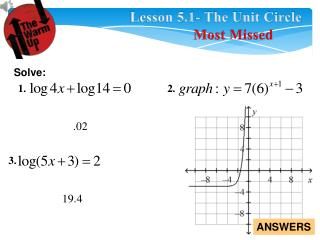# Lesson 5.1- The Unit Circle - PowerPoint PPT PresentationDownload PresentationLesson 5.1- The Unit Circle

Lesson 5.1- The Unit CircleDownload Presentation## Lesson 5.1- The Unit Circle

- - - - - - - - - - - - - - - - - - - - - - - - - - - E N D - - - - - - - - - - - - - - - - - - - - - - - - - - -
##### Presentation Transcript

1. Lesson 5.1- The Unit Circle Most Missed Solve: 1. 2. 3. ANSWERS

2. PRE Lesson 5.1 The Unit Circle • Objectives: • To fill in the circle with degrees and radians. • To find terminal points on the unit circle.

3. Trig Vocabulary

4. What is the circumference Of a circle with a radius of 1?

5. These are called Radians:

6. Trig Vocabulary

7. What would be the degrees of these measures?

8. Relationship between Degrees and Radians To convert degrees to radians, multiply by To convert radians to degrees, multiply by

9. Ex 1. Find the degrees or radians of each:

10. What will be the value measure of these?

11. What will be the degree measure of these?

12. Constructing Sine: Cosine: Tangent:

13. Constructing Sine, Cosine, Tangent:

14. Constructing Sine, Cosine, Tangent:

15. What is the coordinate for this point?

16. Chapter 5 Quiz will be on this:

17. Classwork:Worksheet: 5.1 Unit Circle Worksheet